# Exercise 12.4 Algebraic-Expressions -NCERT Solutions Class 7

## Chapter 12 Ex.12.4 Question 1

Observe the patterns of digits made from line segments of equal length. You will find such segmented digits on the display of electronic watches or calculators.

(a)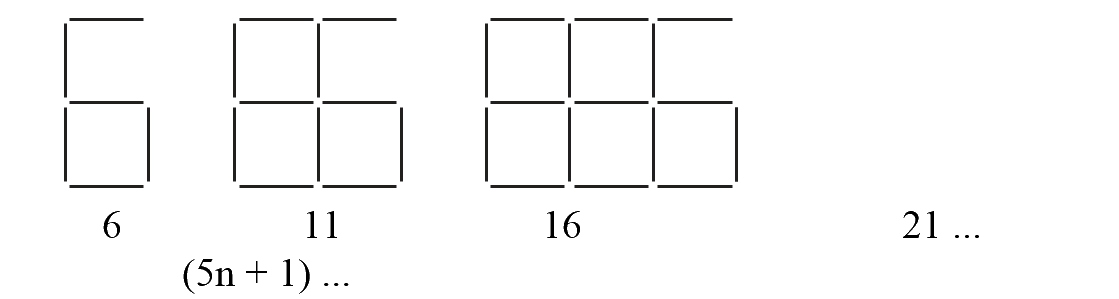(b)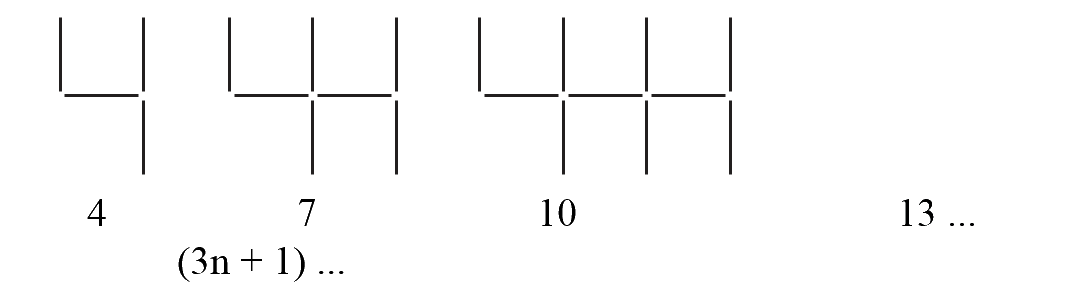(c)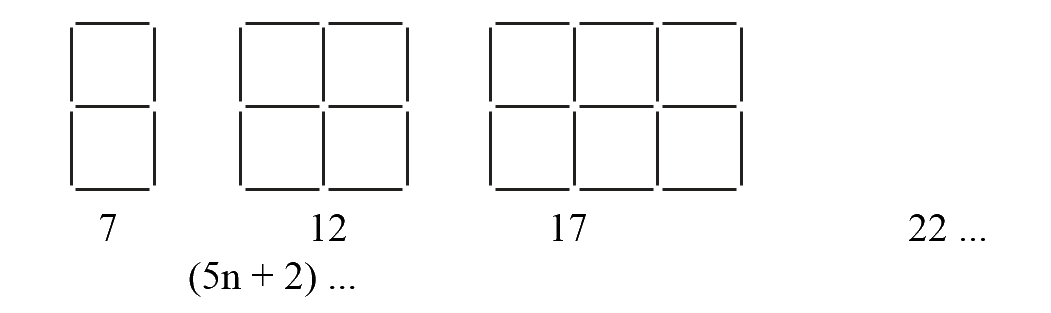If the number of digits formed is taken to be n, the number of segments required to form n digits is given by the algebraic expression appearing on the right of each pattern. How many segments are required to form $$5, 10, 100$$ digits of the kind,### Solution

What is known?

The patterns of digits made from line segments of equal length

What is unknown?

Number of segments required to form $$5, 10, 100$$ digits of the kind, ,Reasoning:

This question is very easy like simply put the value of n in the pattern formulae and you can easily find out the number of segments.

Steps:

Putting value of $$n=5, 10$$ and $$100$$ in the pattern formulae.

$$({\rm{i}})\quad 5n + 1$$ \begin{align}5 \times 5 + 1 & = 25 + 1\\& = 26\\5 \times 10 + 1 & = 50 + 1\\& = 51\\5 \times 100 + 1 & = 500 + 1\\& = 501\end{align}

$${\rm{(ii)}}\quad 3n + 1$$ \begin{align}3 \times 5 + 1 & = 15 + 1\\& = 16\\3 \times 10 + 1 &= 30 + 1\\& = 31\\3 \times 100 + 1 & = 300 + 1\\& = 301\\\end{align}

$$( {{\rm{iii}}}) \quad 5n + 2$$  \begin{align}\\5 \times 5 + 2 & = 25 + 2\\&= 27\\5 \times 10 + 2 & = 50 + 2\\& = 52\\5 \times 100 + 2 & = 500 + 2\\& = 502\\\end{align}

 S. No. Symbol Digit’s Number Pattern’s Formulae No. of. Segments (i)$$5$$ $${\rm{5 n + 1}}$$ 26 $$10$$ 51 $$100$$ 501 (ii)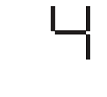$$5$$ $${\rm{3}}\,{\rm{n + 1}}$$ 16 $$10$$ 31 $$100$$ 301 (iii)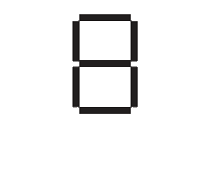$$5$$ $${\rm{5 n + 2}}$$ 27 $$10$$ 52 $$100$$ 502

## Chapter 12 Ex.12.4 Question 2

Use the given algebraic expression to complete the table of number patterns.

 S. No. Expression Terms 1st 2nd 3rd 4th 5th ... 10th … 100th … (i) $$2n – 1$$ $$1$$ $$3$$ $$5$$ $$7$$ $$9$$ - $$19$$ - - - (ii) $$3n+2$$ $$5$$ $$8$$ $$11$$ $$14$$ - - - - - - (iii) $$4n + 1$$ $$5$$ $$9$$ $$13$$ $$17$$ - - - - - - (iv) $$7n + 20$$ $$27$$ $$34$$ $$41$$ $$48$$ - - - - - - (v) $$n^2 +1$$ $$2$$ $$5$$ $$10$$ $$17$$ - - - - $$10,001$$ -

### Solution

What is known?

Different algebraic expressions and the terms.

What is unknown?

Some terms of the given algebraic expression.

Reasoning:

This question is very simple put the value of n in the given algebraic expression and you can easily find out the unknown terms.

Steps:

Putting value of $$n = 5, 10$$ and $$100$$

(i) $$2n – 1$$

\begin{align}2 \times 100 - 1 & = 200 - 1\\& = 199\end{align}

(ii) $$3n+2$$

\begin{align}3 \times 5 + 2 & = 15 + 2\\ & = 17\\\ 3 \times 10 + 2&=30+ 2\\ &= 32 \\ 3 \times 100 + 2 & = 300 + 2\\& = 302\\\end{align}

(iii) $$4n+1$$

\begin{align}4 \times 5 + 1& = 20 + 1\\&= 21\\ 4 \times 10 + 1&= 40 + 1\\ & = 41\\4 \times 100 + 1&= 400 + 1\\ & = 401\end{align}

(iv) $$7n+2$$

\begin{align}7 \times 5 + 20 & = 35 + 20\\&= 55\\7 \times 10 + 20 & = 70 + 20\\&= 90\\7 \times 100 + 20 & = 700 + 20\\&= 720\end{align}

(v) $$n^2+1$$

\begin{align}5 \times 5 + 1 & = 25 + 1\\& = 26\\10 \times 10 + 1 & = 100 + 1\\& = 101\\100 \times 100 + 1 & = 10000 + 1\\& = 10001\end{align}

Complete table is:

 S.No. Expression Terms 1st 2nd 3rd 4th 5th ... 10th … 100th … (i) $$2n – 1$$ $$1$$ $$3$$ $$5$$ $$7$$ $$9$$ - $$19$$ - $$199$$ - (ii) $$3n + 2$$ $$5$$ $$8$$ $$11$$ $$14$$ $$17$$ - $$32$$ - $$302$$ - (iii) $$4n + 1$$ $$5$$ $$9$$ $$13$$ $$17$$ $$21$$ - $$41$$ - $$401$$ - (iv) $$7n + 20$$ $$27$$ $$34$$ $$41$$ $$48$$ $$55$$ - $$90$$ - $$720$$ - (v) $$n^2 + 1$$ $$2$$ $$5$$ $$10$$ $$17$$ $$26$$ - $$101$$ - $$10,001$$ -
Instant doubt clearing with Cuemath Advanced Math Program